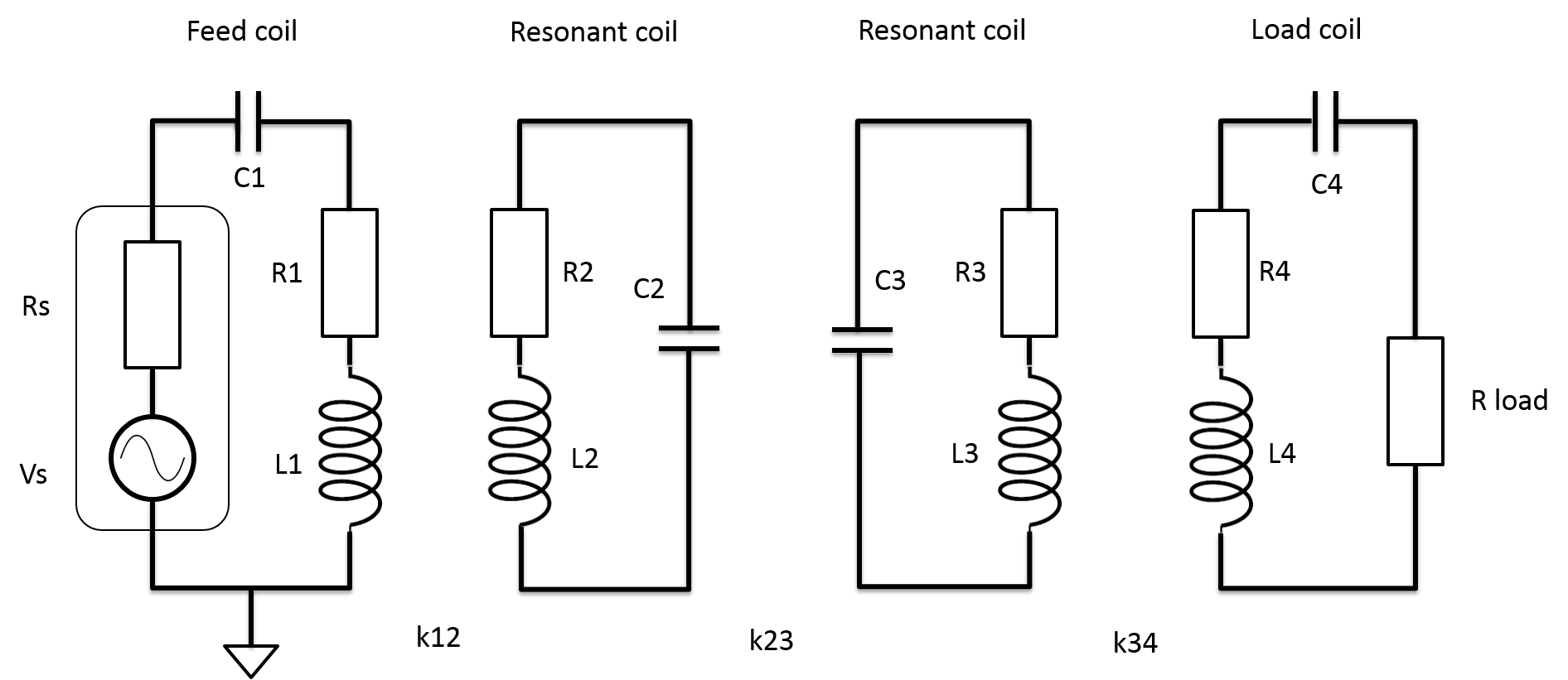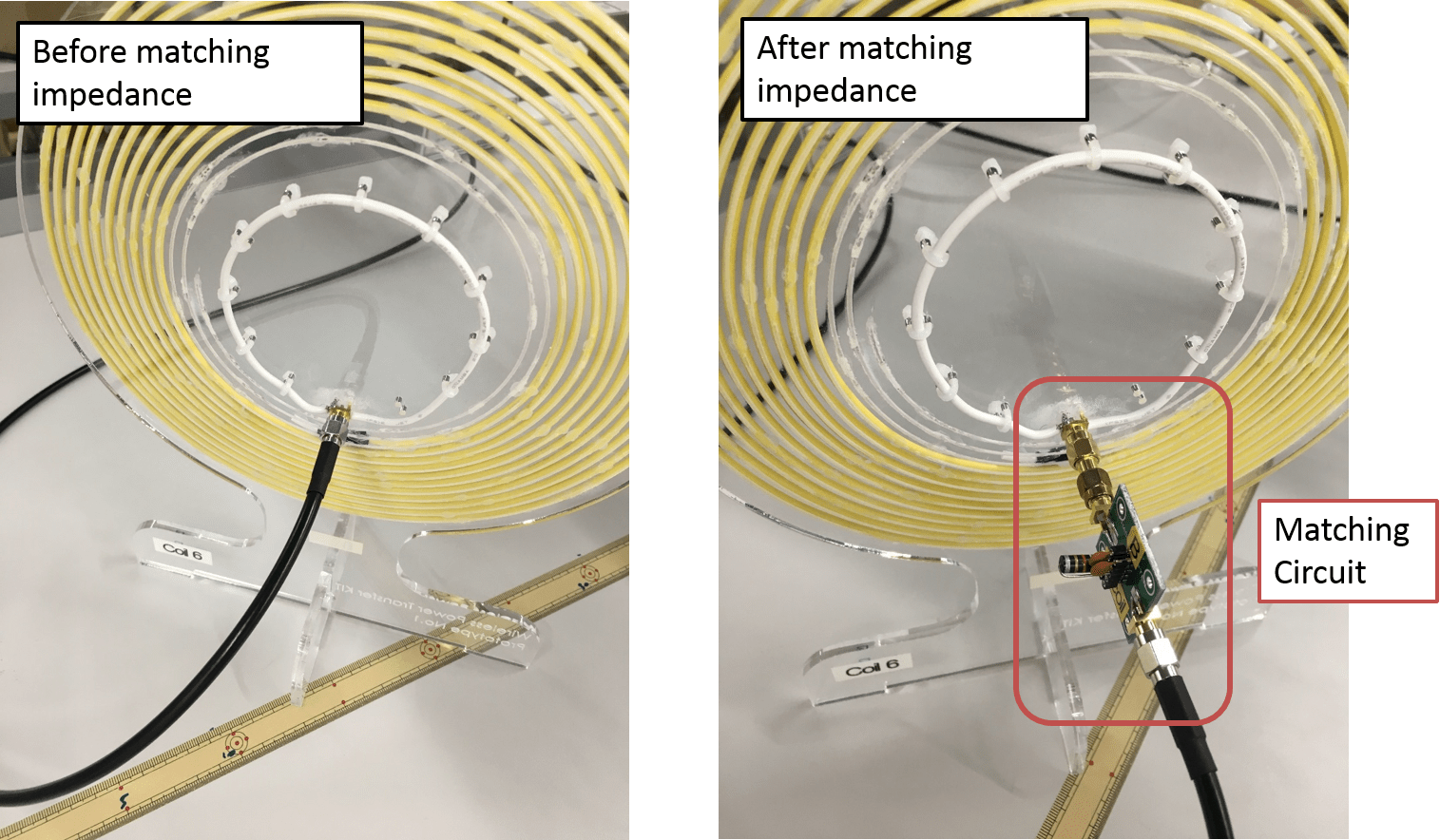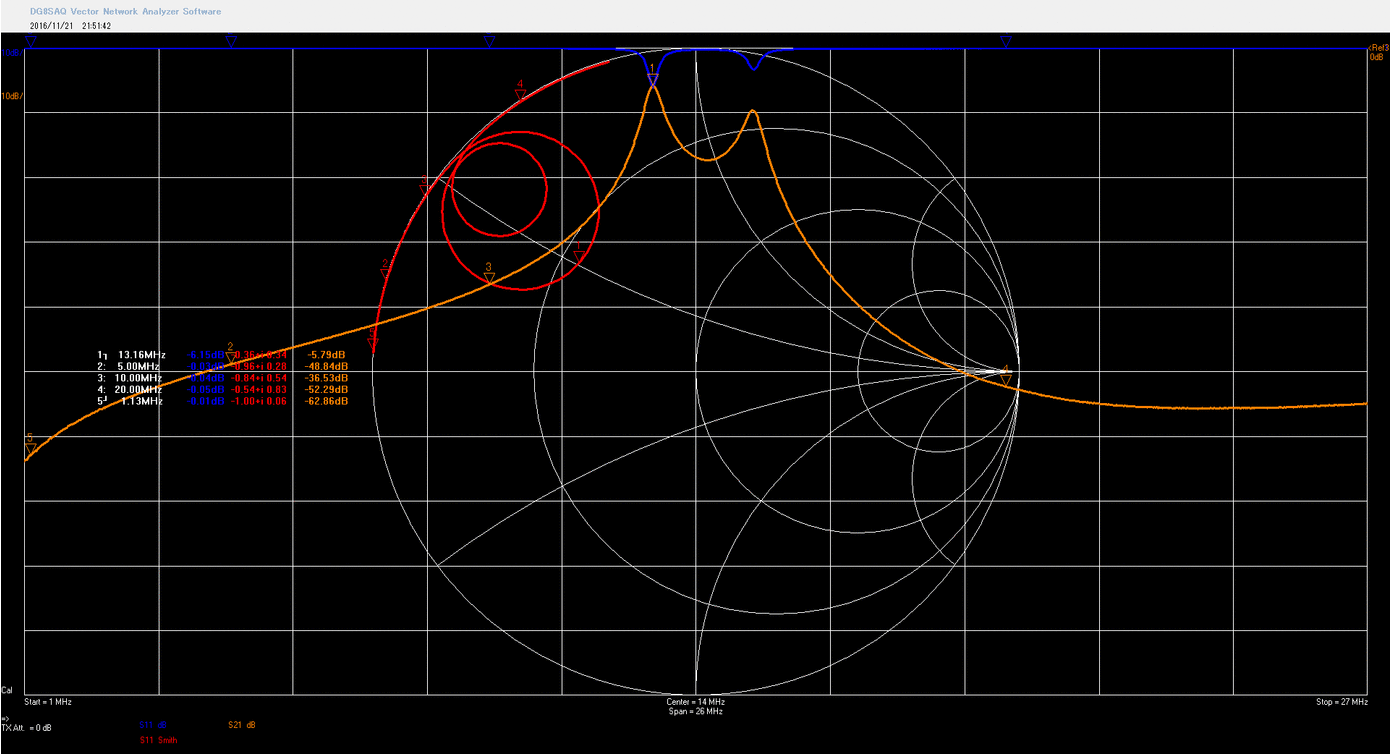Transmission efficiency of the wireless power transfer (WPT) system can be measured with a vector network analyzer (VNWA).

The following picture shows how we measured the transmission efficiency of this system. The yellow WPT coil in the left side is connected to the port 1 of VNWA, while the blue WPT coil in the right side is connected to the port 2 of VNWA. The resonant frequency of this system is set to be 14 MHz. Therefore, the frequency range in this measurement is between 1 MHz to 27 MHz.The following figure shows the equivalent circuit of this WPT coil. Each component’s values were calculated by analyzing the input impedance of WPT coil. 

Rs is the series impedance of the signal source. In this system, Rs should be 50 Ohm. and R load is also 50 ohm because the blue WPT coil is connected to the port 2 of VNWA.We measured the S parameters (S11 : reflection , S21 : transmission and Smith chart) by changing the distance between two resonant coils. The distance are from 10 cm to 75 cm by 5 cm difference.As I mentioned at previous post. the input impedance of WPT coil is not set to be 50 Ohm. In order to match the input impedance to be 50 Ohm, We made a T-network matching circuit. 

In this measurement, We measured S parameters without a matching circuit first, then measured again with a matching circuit. Then we will see how these two different conditions affects the transfer efficiency of this system. The following figure shows the difference between before and after matching impedance of WPT coils. Please look at the SMA connector of feed coils.First, We see the measurement results of un-matched system. This figure showed the change of S11, S21 and Smith chart of this system while distance between two coils changes from 10 cm to 75 cm. The blue line showed the S11 parameter in dB. The yellow line showed the S21 parameter in dB. Smith chart of S11 was expressed by red line.

First, Let’s look how S21 and S11 changes in the resonant frequency (f=14 MHz). While the distance between of two coil is relatively close. the coupling between two resonant coils splits the resonant frequency. That results in low transfer efficiency at the resonant frequency. Because of the regulation of EM equipment, it is not allowed to change the frequency in the WPT machine.

Please look at the cursor No 1.  of the smith chart,  we can see that the input impedance still far from the 50 Ohm at all measurements.Then, We see the measurement results of matched system. This figure showed the change of S11, S21 and Smith chart of this system while distance between two coils changes from 10 cm to 75 cm. The blue line showed the S11 parameter in dB. The yellow line showed the S21 parameter in dB. Smith chart of S11 was expressed by red line.

First, Let’s look how S21 and S11 changes in the resonant frequency (f=14 MHz). While the distance between of two coil is relatively close. the coupling between two resonant coils splits the resonant frequency. However we could see that the S21 value is higher than that of unmatched system at all distance. You could see S11 changes deeper and sharper while the distance becomes larger.

When we look at the cursor No 1.  of the smith chart, We could see the input impedance of TX coil. I expected that input impedance should be matched to be 50 ohm at all distance. However, until distance becomes 50 cm, the slight coupling between two resonant coil will effect the input impedance of coil very much. If we clear this problem in the matching by slight coupling, we could realize 100 % transfer (S21 = 0 [dB]) at some region. This is why we need an adaptive impedance matching system in this inductive resonant WPT system.

Thank you for reading this article.Reference

##### Similar Posts

このサイトはスパムを低減するために Akismet を使っています。コメントデータの処理方法の詳細はこちらをご覧ください